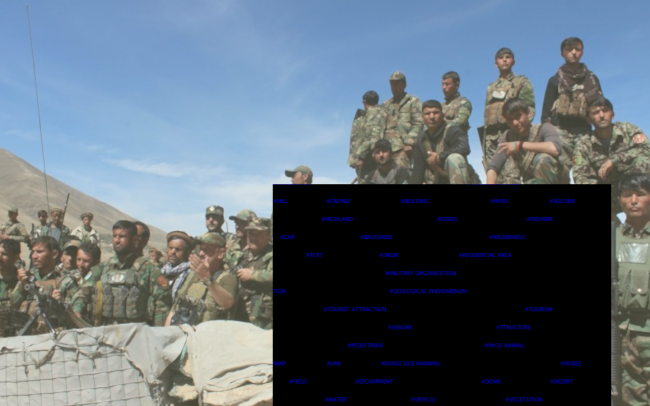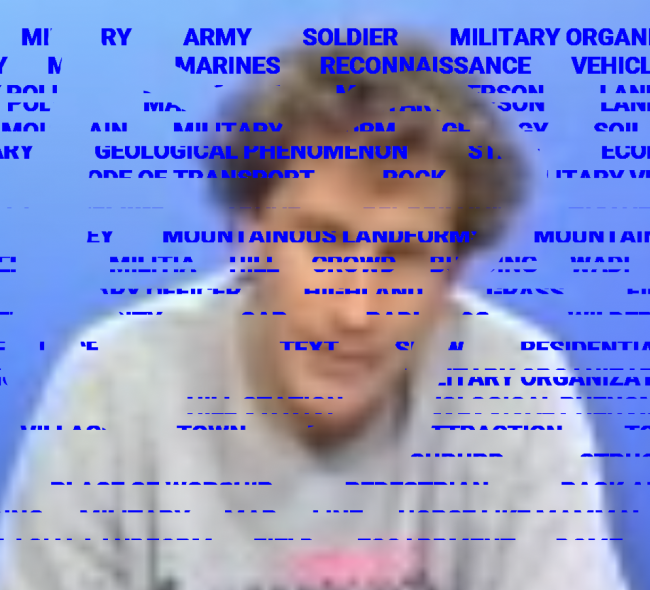#### Howdy, Stranger!

We are about to switch to a new forum software. Until then we have removed the registration on this forum.

# masking PGraphics with PGraphics creates background where it's supposed to be transparent

edited June 2018

I'm trying to mask one PGraphics (that has text) with another (shape) and show the masked text over the "regular" canvas that's showing images.

The masked area becomes entirely opaque (the black rect). I would have used blending mode but I'm not using 100% white or black text.but I see that also that has similar behavior.

``````mask = createGraphics(width, height);
pg = createGraphics(width, height);
for (int x=0; x< labels.length/2; x++){
pg.beginDraw();
pg.fill(0,0,255);
pg.text("#"+labels[x].labelText.toUpperCase(), labels[x].xLoc, labels[x].yLoc);
pg.endDraw();
}
``````

here's the code for the masking:

``````  mask.beginDraw();
mask.rect(random(0,width/2), random(0,height/2), random(width/2, width), random(height/2, height));
image(pg, 0, 0);
``````
Tagged:

• can you print mask.format? i've a feeling it's not rgba, just rgb

runnable examples are better...

• @koogs mask.format print returned "2"

• also manually changing both PGraphics.format to either 0,1,2 made no impact.

• Are you doing this in setup()? Remove `mask.clear();` and see if it works. If you provide an mcve, I will be glad to help further.

Kf

• here I made one custom:

``````PGraphics pg;

float recX = 0;
float recY = 30;
float recW = 30;
float recHigh = 60;

void setup(){
fullScreen();
pg = createGraphics(width, height);
pg.beginDraw();
pg.fill(255,0,0);
for(int i=1; i<20; i++){
pg.text("hello world", random(0,width), random(0,height));
}
pg.endDraw();

}

void draw(){
background(255);

if(frameCount%20 == 0){
recX = random(0,width/2);
recY = random(0,height/2);
recW = random(width/2, width);
recHigh = random(height/2, height);
}

image(pg, 0, 0);

}
``````
• what are we seeing? what are we meant to be seeing?

• In this sample code I'm trying to get masked red text on white background. But the area that's supposed to be unmasked gets a black background. Assuming that the background for the text pgraphics should be transparent so any image on the regular canvas could be seen.

• I don't understand your mask. If you want to write red text on your picture, wouldn't you just draw your picture and then write the text on top of it? No need for masks.

Maybe I am misunderstanding your concept. You want to draw an image, set a rectangle covering that image so the image is hidden. Then you write text in the rectangle. You want to see the image through your text printings over the rectangle?

Kf

• This is a code that demonstrate the second concept above. Check this for reference: https://processing.org/reference/PImage_mask_.html

Kf

``````final color C1=color(250, 0, 0);
final color C2=color(250, 250, 0);

final int SHOWBACKGROUNDONLY=0;
final int SHOWIMAGE=1;

PImage bc;
PGraphics pg;

int state=SHOWBACKGROUNDONLY;

float recX = 0;
float recY = 30;
float recW = 30;
float recHigh = 60;

void setup() {
//fullScreen();
size(600, 400);
pg = createGraphics(width, height);

bc=createImage(width, height, ARGB);
for (int i=0; i<bc.pixels.length; i++) {
bc.pixels[i]=lerpColor(C1, C2, (i%width)*1.0/width);
}
bc.updatePixels();

pg.beginDraw();
pg.background(0);
pg.fill(255);
pg.noStroke();
for (int i=1; i<20; i++) {
pg.text("hello world", random(0, width), random(0, height));
}
pg.ellipse(width/2, height/2, width*0.3, 25);
pg.endDraw();

noLoop();
surface.setTitle("Current state="+state);
}

void draw() {
background(color(0, 102, 153));

if (state==SHOWBACKGROUNDONLY)
return;

image(bc, 0, 0);
}

void mousePressed() {
redraw();

state=(state+1)%3;
surface.setTitle("Current state="+state);
}
``````
• Sorry if I wasn't clear I wish to mask the text layer with rectangles, so only a portion of the text will appear but the background behind the text would be 100% visible.

In the following example (photoshop) I've created text and masked parts of it, I wish to achieve a similar result. When I try to do the same in processing it creates a black background wherever the the mask pgraphic has pixels.• Sounds like you want to copy a rectangular piece of a partially transparent layer over the top of another layer. ???

Will copy() work? There's a version where you can specify the source image and the location and size of the bit you want to copy.

• this creates a text layer full of red numbers on a transparent background and then copies a rectangular section of that onto the grey background.

press mouse to redraw

note that the numbers do not move, but you can only see the ones within the random rectangle.

``````PGraphics textLayer;

int recX, recY;
int recW = 30;
int recH = 60;

void setup(){
size(800, 600, P2D);

// grid of numbers
textLayer = createGraphics(width, height);
textLayer.beginDraw();
textLayer.clear();  // transparent
textLayer.fill(255, 0, 0);
int i = 0;
for(int y = 0; y < height; y += 50){
for(int x = 0; x < width; x += 50){
textLayer.text(i, x, y);
i++;
}
}
textLayer.endDraw();

randomise();
}

void draw(){
background(128);

copy(textLayer, (int)random(textLayer.width), (int)random(textLayer.height), recW, recH,
(int)random(width), (int)random(height), recW, recH);

noLoop();
}

void mousePressed() {
randomise();
loop();
}

void randomise() {
recX = (int)random(width / 2);
recY = (int)random(height / 2);
recW = (int)random(width/2, width);
recH = (int)random(height/2, height);
}
``````
• My attempt using JAVA2D renderer instead of P2D, extending from @koogs. There is only one small difference when calling the `copy()` function.

Kf

``````final color C1=color(0, 20, 220);
final color C2=color(250, 250, 0);

PGraphics textLayer;
PImage bc;

int recX, recY;
int recW = 30;
int recH = 60;

void setup() {
size(800, 600);

// grid of numbers
textLayer = createGraphics(width, height);
textLayer.beginDraw();
textLayer.textSize(16);
textLayer.clear();  // transparent
textLayer.fill(255, 0, 0);
int i = 0;
for (int y = 0; y < height; y += 50) {
for (int x = 0; x < width; x += 50) {
textLayer.text(i, x, y);
textLayer.text(i, x+1, y);  //Offset by 1px to create bold effect
i++;
}
}
textLayer.endDraw();

//Background img
bc=createImage(width, height, ARGB);
for (int k=0; k<bc.pixels.length; k++) {
bc.pixels[k]=lerpColor(C1, C2, (k%width)*1.0/width);
}
bc.updatePixels();

randomise();
}

void draw() {
background(bc);

copy(textLayer.get(), (int)random(textLayer.width),
(int)random(textLayer.height),
recW,
recH,
(int)random(width), (int)random(height), recW, recH);

noLoop();
}

void mousePressed() {
randomise();
loop();
}

void randomise() {
recX = (int)random(width / 2);
recY = (int)random(height / 2);
recW = (int)random(width/2, width);
recH = (int)random(height/2, height);
}
``````
• I think you need to use the same position for both the source and the destination so the text layer is always aligned with the background. The bit of the text layer you see is different each time but the text is always in the same absolute position.

• oh wow, this is exactly what I needed how come the regular .mask() didn't behave this way and regarded the masked layer's original alpha values of the visible area?